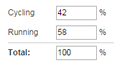# Continuous SumView live demo

A Continuous Sum question contains a series of linked text fields, but the entry is restricted to numberic values only (numbers). When respondents type into these fields, the system can display a running total at the bottom. You can also use validation to ensure that the total equals a certain value, for example you could ensure that all percentage values entered total 100%.

Continuous Sums are ideal when you want to calculate quantities, time or expenses within your survey. By adding a prefix and/or a suffix, the respondent can easily understand the format that you expect in each field.

## Creating Continuous Sum Questions

To create your Continuous Sum question:

2. Type your question in the Question Text field.
3. In the Question Type drop-down menu, select Continuous Sum.
4. In the Answer Choices field, type the text that will be displayed on the left-hand side of each field.
5. Scroll down to the Display Options section.
• In the Answer Prefix field, type the character you would like to display before the field.
• In the Answer Suffix field, type the character you would like to display after the field.
• Check the Display Total Sum checkbox to display a running total for all of the fields in the question.
• In the Total Row Label field, type any text that will appear before the running total if Display Total Sum is checked.
6. Scoll down to the Validation Options section.
• Choose the Answer Format that you require: Whole Number or Decimal Number.
• Check the Require a Fixed Sum checkbox to ensure that the data in the fields adds up to a valid figure. (For example, when collecting percentages, your Fixed Sum would be 100, to ensure that the respondent's answers add up to 100 percent. When asking a respondent to enter all minutes in an hour, your Fixed Sum would be 60.)